## 8月24日更新

• 修复苹果cms程序根目录有api.php文件的问题
• 增加php7.X版本代码。

## 酷Q机器人

• 精简代码，小巧灵活：精简大量不必要代码，减小酷Q体积，优化程序速度，使得酷Q更加轻巧好用
• 多线处理，瞬间完成：程序全部采用多线程处理消息，每条消息不互干扰，瞬间完成处理及发送。
• 插件扩展，功能多样：包含强大的插件机制，可以安装各式各样的插件，扩展酷Q的各项功能。
• 掉线检测，崩溃防护：掉线重登检测，保持长期在线。代码严格审查，最大限度减少异常崩溃。

## 要求

• PHP版本为5.X。7.X也支持。7.X的请自行修改代码
• 适用苹果V10。苹果V8的表名与苹果V10表名不同，请自行修改。

## 登录软件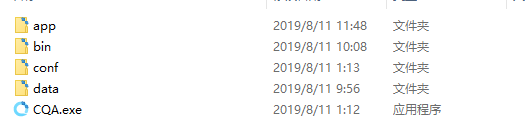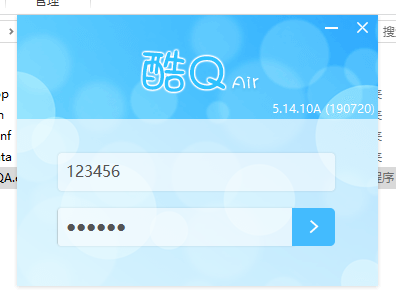## 下载并使用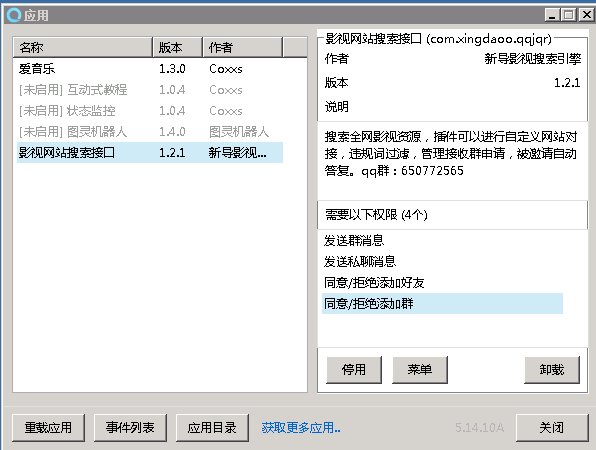• 主人QQ：可以使用主人QQ对qq机器人进行简单的设置，无需借助软件设置。
• 触发搜索词：设置搜索词为“搜”，在群里发送消息如：搜陈情令，会自动触发该脚本并为寻找陈情令的结果地址。
• 搜索结果前/后缀：就是在机器人每说一段话之前/之后加内容。
• 搜索结果为空时：就是什么也没搜索到的时候返回的信息。如果你设置了搜索结果前后缀，在这里填写内容是无法返回的，除非你的视频站服务器出故障无响应。
• 开启自定义网站：默认不勾选。一定要勾选，不然机器人发送的信息都是别人的。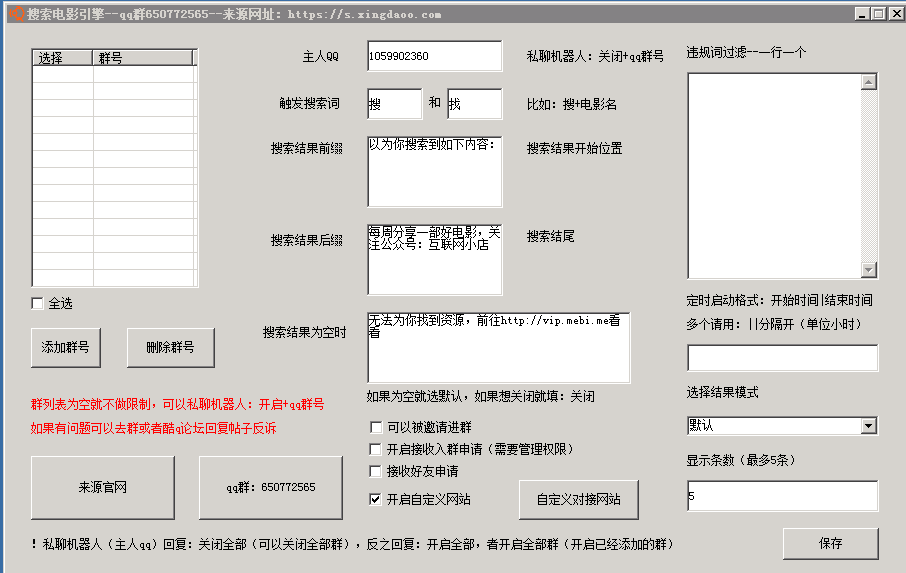## 自定义网站

### PHP5.X版本

<?php
//db_name修改成你用户名，db_psd修改成你数据库登录密码
$con = mysql_connect("localhost","db_user","db_psd"); mysql_query("SET NAMES UTF8"); mysql_query("set character_set_client=utf8"); mysql_query("set character_set_results=utf8"); //请把mac修改成你苹果数据库的名称 mysql_select_db("mac",$con);
$sql = "SELECT * FROM mac_vod WHERE vod_name like '%".$_GET["so"]."%' LIMIT 0 , 10";
$result = mysql_query($sql,$con);$i = 1;
$r = ""; while($row = mysql_fetch_array($result)) { //把vip.mebi.me/vod/detail-务必修改为你的网站的视频详情页地址格式！$r .= '<a class="vodurl" href="http://vip.mebi.me/vod/detail-'.$row['vod_id'].'"><span class="vodname">'.$i.'、'.$row['vod_name'].$row['vod_remarks']."</spa></a><br />";
$i++; } if ($r)
{
$r = '<div class="result">'.$r.'</div>';
}else{
//请把http://vip.mebi.me修改为你苹果CMS站点网址
$r = '<div class="result"><a class="vodurl" href="http://vip.mebi.me"><span class="vodname">没有找到资源，请注意片名是否书写正确。片名正确仍提示没有找到资源，则全网暂时未上线该视频，建议过几天再来查找。</span></a><br /></div>'; } echo$r;
?>


### PHP7.X版本

<?php
//db_user修改为你的用户名，db_psd修改成你数据库登录密码，db_name修改成你数据库的名称
$con = mysqli_connect("localhost","db_user","db_psd","db_name"); mysqli_query($con, "SET NAMES UTF8");
mysqli_query($con, "set character_set_client=utf8"); mysqli_query($con, "set character_set_results=utf8");
$sql = "SELECT * FROM mac_vod WHERE vod_name like '%".$_GET["so"]."%' LIMIT 0 , 10";
$result = mysqli_query($con,$sql);$i = 1;
$r = ""; while($row = mysqli_fetch_array($result)) { //把vip.mebi.me/vod/detail-务必修改为你的网站的视频详情页地址格式！$r .= '<a class="vodurl" href="http://vip.mebi.me/vod/detail-'.$row['vod_id'].'"><span class="vodname">'.$i.'、'.$row['vod_name'].$row['vod_remarks']."</span></a><br />";
$i++; } if ($r)
{
$r = '<div class="result">'.$r.'</div>';
}else{
//请把http://vip.mebi.me修改为你苹果CMS站点网址
$r = '<div class="result"><a class="vodurl" href="http://vip.mebi.me"><span class="vodname">没有找到资源，请注意片名是否书写正确。片名正确仍提示没有找到资源，则全网暂时未上线该视频，建议过几天再来查找。</span></a></div>'; } echo$r;
?>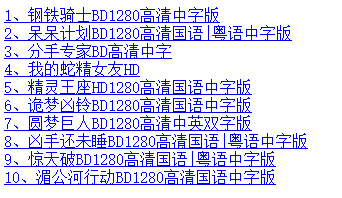• 域名：你的域名
• 搜索页：/sql.php?so=
• 请求方式：GET
• 要提取的范围：<div class="result">
• 提取范围结尾：</div>
• 提取标题开始：<span class="vodname">
• 标题结尾：</span>
• 提取网址开始：<a class="vodurl" href="
• 网址结尾：">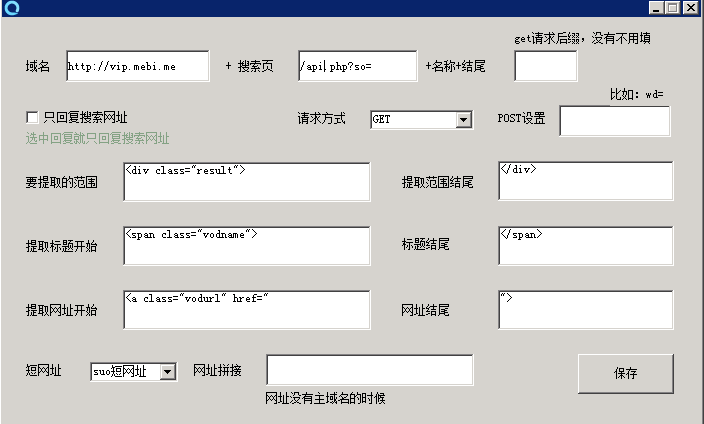" title="">

## 插件下载

g1bz

Last modification：December 16th, 2020 at 02:16 pm